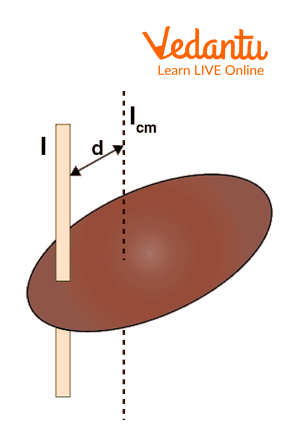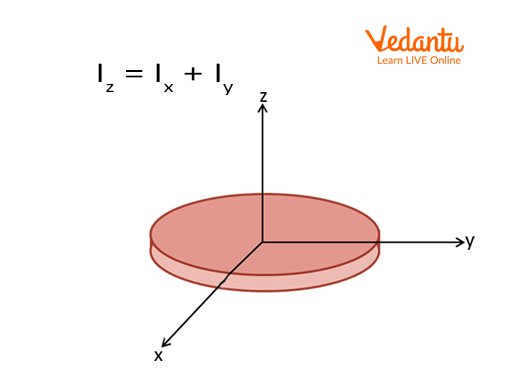Courses
Courses for Kids
Free study material
Offline Centres
More

# Moment of Inertia of Continuous Bodies - JEE Important TopicLast updated date: 28th Nov 2023
Total views: 21k
Views today: 0.21k## What is the Moment of Inertia?

The moment of inertia is a measure of an object's resistance to changes in its rotation. It is the rotational analogue to mass, which quantifies an object's resistance to changes in its linear motion. The moment of inertia depends on the shape of the object and its distribution of mass. For example, a sphere has a lower moment of inertia than a cylinder with the same mass and radius because the sphere's mass is more evenly distributed around its centre.

The moment of inertia is important in many fields, including Engineering, Physics, and Astronomy. In Engineering, it is used to calculate the strength of beams and columns. In Physics, it is used to study the dynamics of rotating objects.

## Formula of Moment of Inertia

The formula of moment of inertia (I) is the sum of the products of the mass (m) of each particle and the square of its distance (r) from the axis of rotation:

$\Rightarrow l=m{{r}^{2}}$

This equation is derived from Newton's second law of motion, which states that the sum of the forces acting on a body is equal to its mass times its acceleration. The formula can be used to calculate the moment of inertia for any object, provided that the masses and distances of all particles are known.

## Factors on which Moment of Inertia Depends

There are three primary factors which affect the moment of inertia of an object. They are:

• The distribution of mass within the object - How the mass is distributed will affect how much rotational force is required to move it.

• The shape of the object - A more compact shape will have a lower moment of inertia than a less compact one.

• The size of the object - A larger object will have a higher moment of inertia than a smaller one.

## Moment of Inertia of Continuous Bodies Depends on

The moment of inertia of a continuous body is the sum of the moments of inertia of all its constituent parts. The total moment of inertia is a measure of an object's resistance to changes in its rotation. A body with a large moment of inertia will require more force to change its rotation than a body with a small moment of inertia.

The moment of inertia of a continuous body depends on the distribution of mass within that body. If the body is symmetrical, then the moment of inertia will be lower than if it were not symmetrical. For example, a sphere has a lower moment of inertia than an ellipsoid. The reason for this is that the mass of a sphere is evenly distributed throughout its volume, while the mass of an ellipsoid is not.

The second factor that affects the moment of inertia of a continuous body is the shape of that body. A slender body has a lower moment of inertia than a squat body. This is because a slender body has less mass at its edges, where it would rotate if it were to fall over.

Finally, the third factor affecting the moment of inertia of a continuous body is the location of its centre of gravity. A body with its centre of gravity closer to its edges will have a higher moment of inertia than one with its centre of gravity closer to its centre. This is because when a body rotates, all of its mass does not rotate around the centre of gravity; only the mass at its edges does so.

## Theorems to Calculate Moment of Inertia of Bodies

If we observe the moment of inertia of different bodies then we will get to know that the position of the axis of rotation affects the moment of inertia of bodies. To find the moment of inertia through any given axis, we have theorems which work perfectly for our use case.

## Parallel Axis Theorem

The Parallel Axis Theorem of Moment of Inertia states that the moment of inertia of a body about any axis is equal to the sum of the moments of inertia about a parallel axis through the centre of mass plus the product of the mass and square of the distance between the axes. In other words, this theorem allows us to calculate the moment of inertia for a body about an axis that is not passing through its centre of gravity. This can be very useful when dealing with objects that have irregular shapes or are not symmetrical.Parallel Axis Theorem

The moment of inertia using parallel axis theorem is given by

$\text{l }=\text{ }{{\text{l}}_{cm}}\text{ }+\text{ }M{{d}^{2}}$

Where d is the distance between the two axes.

M is mass of body

${{l}_{cm}}$ is moment of inertia with respect to the centre of mass

## Perpendicular Axis Theorem

The Perpendicular Axis Theorem of Moment of Inertia states that the moment of inertia of any object about an axis perpendicular to its plane of symmetry is equal to the sum of the moments of inertia about any two other mutually perpendicular axes lying in that plane, each passing through the point where the first axis intersects the plane.

${{I}_{z}}\text{ }=~\text{ }{{I}_{x}}\text{ }+~\text{ }{{I}_{y}}$Perpendicular Axis Theorem

## Moment of Inertia for Different Bodies

 Sl. No Shape of Object M.I along the Symmetry Axis M.I about the Diameter 1. Rod $I=\dfrac{1}{12}M{{L}^{2}}$ $I=\dfrac{1}{3}M{{L}^{2}}$ 2. Cylinder $I=\dfrac{1}{2}M{{R}^{2}}$ $I=\dfrac{1}{4}M{{R}^{2}}+\frac{1}{12}M{{L}^{2}}$ 3. Hoop $I=\dfrac{1}{2}M{{R}^{2}}$ $M{{R}^{2}}$ 4. Rectangular Objects ${{I}_{c}}=\dfrac{1}{12}m({{h}^{2}}+{{w}^{2}})$ ${{I}_{d}}=\dfrac{1}{12}m({{h}^{2}}+{{w}^{2}})$ 5. Sphere $I=M{{R}^{2}}$ $I=\dfrac{M{{r}^{2}}}{2}$

## Summary

The moment of inertia is the rotational analogue to mass, which quantifies an object's resistance to changes in its linear motion. It is important in many fields, including Engineering, Physics, and Astronomy. Moment of inertia depends on the shape, size and distribution of mass of an object. A larger or more compact object will require more force to change its rotation than a smaller one. The moment of inertia of a continuous body depends on the distribution of mass within that body.

If the body is symmetrical, then its inertia will be lower than if it is not. This is because the mass of a spherical body is evenly distributed throughout its volume, and an ellipsoid has less mass at its edges. The location of a body's centre of gravity is also affected by the shape of that body - a slender body has a lower moment of stability than a squat one.

## FAQs on Moment of Inertia of Continuous Bodies - JEE Important Topic

1. Does the moment of inertia of a rigid body change with the speed of rotation?

The moment of inertia of a rigid body is determined solely by the distribution of mass about the axis of rotation and is independent of the rotational speed. As a result, the moment of inertia of a rigid body does not change with rotational speed.

2. Why do we calculate the moment of inertia?

Moment of inertia acts as an important tool in rotational motion. For any object or body subjected to rotational motion, the amount of torque required by an item to achieve a given angular acceleration depends on its moment of inertia. The moment of inertia of rigid bodies help us in determining few parameters, such as how to calculate torque or rotational force.